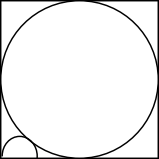Your Insider Guide to the GRE

[not sponsored or endorsed by the ETS]

# Free GRE Test Questions

Question 1 of 10
ID: GRE-MC-1
Section: Quantitative Reasoning - Multiple Choice with one answer
Topics: Geometry; Circles
Difficulty level: Challenging

(Practice Mode: Questions of Section » Back to Overview)

In the square shown above, the side is 2 units. The circle and the semicircle having its diameter along a side of the square, touch as shown. What is the radius of the smaller semicircle?A$\frac{1}{4}$
B$\frac{1}{2\sqrt{2}}$
C$\sqrt{2}-1$
D$\frac{1}{\sqrt{2}}$
E$\frac{1}{2}$